Courses

# Ex 7.2 NCERT Solutions- Coordinate Geometry Class 10 Notes | EduRev

## Class 10 : Ex 7.2 NCERT Solutions- Coordinate Geometry Class 10 Notes | EduRev

The document Ex 7.2 NCERT Solutions- Coordinate Geometry Class 10 Notes | EduRev is a part of the Class 10 Course Mathematics (Maths) Class 10.
All you need of Class 10 at this link: Class 10

Q.1. Find the coordinates of the point which divides the join of (-1, 7) and (4, -3) in the ratio 2 : 3.
Sol. Let P(x, y) be the point.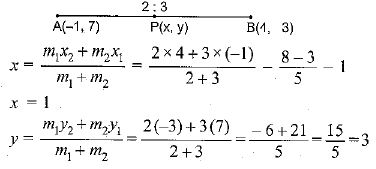y = 3
Then, the coordinates of point are (1,3)

Q.2. Find the coordinates of the points of trisection of the line segment joining (4, -1) and (-2, -3).
Sol.  Let points P and Q trisect the line joining the points.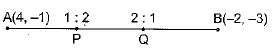∴ AP = PQ = QB
P divides AB in the ratio 1:2 and Q divides AB in the ratio 2:1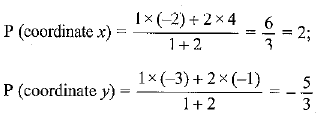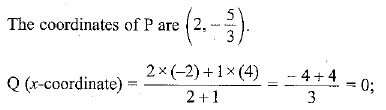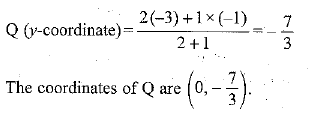Q.3. To conduct Sports Day activities, in your rectangular shaped school ground ABCD, lines have been drawn with chalk powder at a distance of 1 m each. 100 flower pots have been placed at a distance of 1 m from each other along AD, as shown in the figure. Niharika runs 1/4 th the distance AD on the 2nd line and posts a green flag. Preet runs 1/5 th the distance AD on the eighth line and posts a red flag. What is the distance between both the flags? If Rashmi has to post a blue flag exactly halfway between the line segment joining the two flags, where should she post her flag?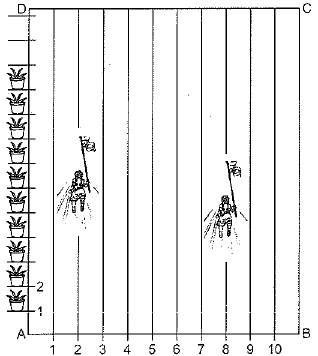Sol.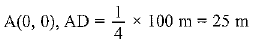Coordinates of green flag are P(2, 25)
The coordinates of red flag are Q(8, 20)
The distance between two points is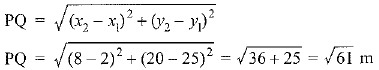Position of the blue flag = mid-point of PQ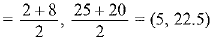∴ The blue flag is in the 5th line, at a distance of 22.5 m.

Q.4. Find the ratio in which the line segment joining the points (-3, 10) and (6, -8) is divided by (-1, 6).
Sol. Let the required ratio be k : 1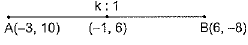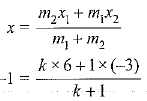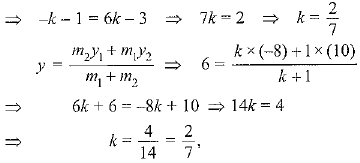∴ The required ratio is 2:7.

Q.5. Find the ratio in which the line segment joining A (1, -5) and B (-4, 5) is divided by the x-axis. Also find the coordinates of the point of division.
Sol. Let P(x, 0) divide the line segment AB in the ratio k : 1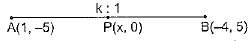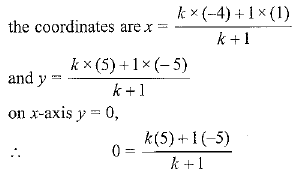0 = 5k - 5 ⇒ 5k = 5 k = 1
Hence the required ratio is 1 : 1, at point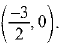Q.6. If (1, 2), (4, y), (x, 6) and (3, 5) are the vertices of a parallelogram taken in order, find x and y.
Sol.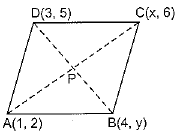Mid-point of AC = Mid-point of BD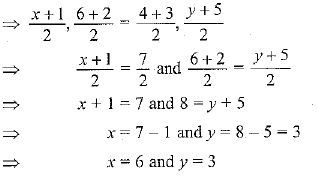Q.7. Find the coordinates of a point A, where AB is the diameter of a circle whose centre is (2, -3) and B is (1, 4).
Sol. Let the coordinates of A be (x, y). O (2, - 3) is the mid-point of AB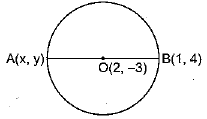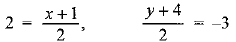⇒ x + 1 = 4, ⇒ y + 4 = -6
⇒ x = 4 - 1, ⇒ y = -6 - 4
⇒ x = 3, ⇒ y = -10
Hence, the coordinates of A are (3, -10).

Q.8. If A and B are (-2, -2) and (2, -4), respectively, find the coordinates of P such that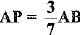and P lies on the line segment AB.
Sol.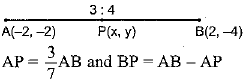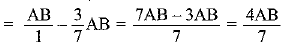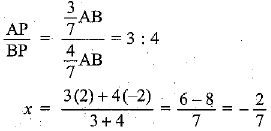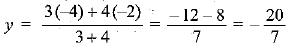Hence, the coordinates of P are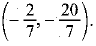Q.9. Find the coordinates of the points which divide the line segment joining A (-2, 2) and B (2, 8) into four equal parts.
Sol. P, Q, R divide AB into four equal parts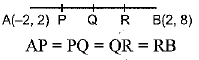Now, Q is the mid-point of AB.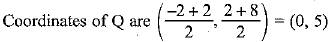P is the mid-point of AQ, then the coordinates of P are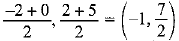R is the mid-point of QB, the coordinates of R are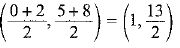Q.10. Find the area of a rhombus if its vertices are (3, 0), (4, 5), (-1, 4) and (-2, -1) taken in order. [Hint: Area of a rhombus = 1/2 (product of its diagonals)]
Sol. Let points be A (3,0), B(4, 5), C(-1, 4) and D(-2, -1)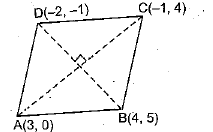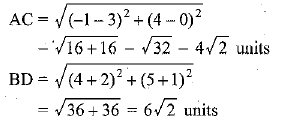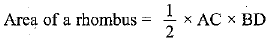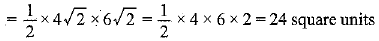Offer running on EduRev: Apply code STAYHOME200 to get INR 200 off on our premium plan EduRev Infinity!

## Mathematics (Maths) Class 10

178 videos|268 docs|103 tests

,

,

,

,

,

,

,

,

,

,

,

,

,

,

,

,

,

,

,

,

,

;